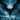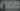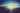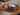Navigate back to the homepage

# 高性能排序算法LER0ever
April 5th, 2018 · 1 min read• 传统的快排，堆排，归并
• 稍微现代的混合(启发)/并行排序。

### 算法列表以及效率

 排序算法 最好时间 平均时间 最坏时间 辅助空间 归并排序 $O(n log n)$ $O(n log n)$ $O(n log n)$ $O(n)$ 堆排序 $O(n log n)$ $O(n log n)$ $O(n log n)$ $O(1)$ 快速排序 $O(n log n)$ $O(n log n)$ $O(n^2)$ $O(log n) ~ O(n)$ 自省排序 $O(n log n)$ $O(n log n)$ $O(n log n)$ - PDQSort $O(n)$ $O(n log n )$ $O(n log n)$ - K路归并 - $O(nklog k)$ or $O(nk^2)$ - - 并行归并 $O((log n)^2)$ $O((log n )^2)$ $O((log n)^3)$ -

## 简单排序算法比较

### 1. 归并排序

#### 原理

1. 假设有两个有序序列，新开一个数组大小为两序列之和，用于存放合并后的序列。
2. 两个指针分别初始指向两序列的起始值
3. 比较两个指针指向的值，将较小的并入结果序列，并移动指针至序列下一个数
4. 重复，直至某一个指针到达了该序列末端
5. 将剩下的序列追加到结果序列

#### 时空复杂度

 最好时间 最坏时间 平均时间 辅助空间 $O(n log n)$ $O(n log n)$ $O(n log n)$ $O(n)$

### 2. 堆排序

#### 原理

1. 构造最大堆
2. 将目标数加入堆末
3. 最大堆性质被破坏，使用沉降法调整最大堆(Reheapify)

#### Reheapify

1. 同样适用分治法，给定两个已经满足最大堆性质的堆，以及一个可能不合适的根节点，执行递归操作
2. 比较根节点和左右子节点，挑一个最大的，如果根节点已经是最大，那么整个堆就是最大堆
3. 否则交换根节点和最大直接点，在该子树总继续调用Reheapify

#### 时空复杂度

 最好时间 最坏时间 平均时间 辅助空间 $O(n log n)$ $O(n log n)$ $O(n log n)$ $O(1)$

### 3. 快速排序

#### 原理

1. 选一个Pivot元素P，将数组分为两个区间，左区间内的所有数都小于等于P，右区间都大于等于P
2. 向左右区间分别递归，同样用执行步骤1.
3. 重复处理，直至区间处理完毕

#### P的选择

##### 比赛中的P选择方法
1. 通常竞赛中的处理方法是随机化选择P，取个A[rand(0…n-1)]作为P处理。 好处就是不怎会退化，坏处就是如果数组特别大，随机数生成开销很大的情况下速度会受很大影响。
2. 还有就是三个数取中位数的方法选择P (Median of three)， 取三个数（可以前三个，或任意三个），找出中位数把它当P
3. 在我们学校的算法课上教授还讲了一种两个维度求中位数已得到非常接近中位数的方法， 不过我还没有实际测试性能。
##### 曲线规避P的问题
1. DualPivotQuickSort，取两个Pivot，实现快速三向切分的快速排序， 例如JDK1.8中的DualPivotQuicksort
2. 元素数量较少的时候，快速排序性能比插入排序差， 所以元素数量低于某个阈值的时候采用插入排序

#### 时空复杂度

 最好时间 最坏时间 平均时间 辅助空间 $O(n log n)$ $O(n^2)$ $O(n log n)$ $O(log n) ~ O(n)$

## 混合/并行排序

### 一. Introsort (std::sort) 内省排序

Introspective Sort 内省式排序是David R. Musser于1996年提出一种混合式排序算法。 大部分情况下特征和Median-of-3 QuickSort类似，但是当分割的结果有恶化倾向时， Introsort能够自我侦测并且切换到堆排序上，使之效率维持在O(n log n)， 并且速度优于从一开始就用堆排序。

std::sort中的Introsort是以上Introsort的改良版本，因为之前提到，在数据量比较小的情况下， 快速排序的递归代价太大，效率还不如插入排序，所以在GNU libstdc++中的 std::sort又被极限优化了：std::sort根据数据量大小以及是否退化将会自动选择采用 快速排序, 堆排序, 插入排序三者之一确保性能最大化。

#### 原理

1. 数据量足够大且足够随机时采用快速排序，此时效率为O(log n)
2. 假设一旦某次分段后数据量小于一定的阈值，改用插入排序处理该段数据，此时效率可能达到O(n)
3. 如果递归过程中发现递归层数过深或者分割行为有恶化倾向的时候，能够自动侦测并且换到堆排序，维持效率在O(n log n)

#### 源码分析

##### std::sort 函数主体
.css-1chxjt6{position:absolute;right:22px;top:24px;padding:8px 12px 7px;border-radius:5px;color:#6f7177;-webkit-transition:background 0.3s ease;transition:background 0.3s ease;}.css-1chxjt6:hover{background:rgba(255,255,255,0.07);}.css-1chxjt6[data-a11y="true"]:focus::after{content:"";position:absolute;left:-2%;top:-2%;width:104%;height:104%;border:2px solid var(--theme-ui-colors-accent,#6166DC);border-radius:5px;background:rgba(255,255,255,0.01);}@media (max-width:45.9375em){.css-1chxjt6{display:none;}}1template <class RandomAccessIterator>2inline void sort(RandomAccessIterator first, RandomAccessIterator last) {3    if (first != last) {4        __introsort_loop(first, last, value_type(first), __lg(last - first) * 2);5        __final_insertion_sort(first, last);6    }7}

##### 主函数
1template <class RandomAccessIterator, class T, class Size>2void __introsort_loop(RandomAccessIterator first,3                      RandomAccessIterator last, T*,4                      Size depth_limit) {5    while (last - first > __stl_threshold) {6        if (depth_limit == 0) {7            partial_sort(first, last, last);8            return;9        }10        --depth_limit;11        RandomAccessIterator cut = __unguarded_partition12          (first, last, T(__median(*first, *(first + (last - first)/2),13                                   *(last - 1))));14        __introsort_loop(cut, last, value_type(first), depth_limit);15        last = cut;16    }17}

##### 分割算法
1template <class RandomAccessIterator, class T>2RandomAccessIterator __unguarded_partition(RandomAccessIterator first, 3                                           RandomAccessIterator last, 4                                           T pivot) {5    while (true) {6        while (*first < pivot) ++first;7        --last;8        while (pivot < *last) --last;9        if (!(first < last)) return first;10        iter_swap(first, last);11        ++first;12    }13}

##### 堆排部分排序
1template <class RandomAccessIterator, class T, class Compare>2void __partial_sort(RandomAccessIterator first, RandomAccessIterator middle,3                    RandomAccessIterator last, T*, Compare comp) {4    make_heap(first, middle, comp);5    for (RandomAccessIterator i = middle; i < last; ++i)6        if (comp(*i, *first))7            __pop_heap(first, middle, i, T(*i), comp, distance_type(first));8    sort_heap(first, middle, comp);9}1011template <class RandomAccessIterator, class Compare>12inline void partial_sort(RandomAccessIterator first,13                         RandomAccessIterator middle,14                         RandomAccessIterator last, Compare comp) {15    __partial_sort(first, middle, last, value_type(first), comp);16    }

##### 最终的插入排序
1template <class RandomAccessIterator>2void __final_insertion_sort(RandomAccessIterator first, 3                            RandomAccessIterator last) {4    if (last - first > __stl_threshold) {5        __insertion_sort(first, first + __stl_threshold);6        __unguarded_insertion_sort(first + __stl_threshold, last);7    }8    else9        __insertion_sort(first, last);10}

#### 时空复杂度

 最好时间 最坏时间 平均时间 辅助空间 $O(n log n)$ $O(n log n)$ $O(n log n)$ $O(log n)$

### 二. Pattern-defeating Quicksort

PDQSort是目前单线程排序中最快的了(当然有更快的请指出)。 即使面对GNU libc++里的std::sort各种神优化，pdqsort仍然显著快于std::sort， 并且最快排序复杂度能够到O(n)

#### 原理pdqsort采用的Block Partitioning来自最近的一篇论文Block Quicksort

pdqsort在严格上升或下降或清一色数组时排序复杂度只有O(n)

#### 实现

 Language Sort Implementation C quicksort C++ introsort D introsort Swift introsort Go introsort Crystal introsort Java dual-pivot quicksort Rust pdqsort

#### 时空复杂度

 最好时间 最坏时间 平均时间 辅助空间 $O(n)$ $O(n log n)$ $O(n log n)$ $O(log n)$

### 三. k路归并

#### 原理

1/*2 * main.c3 *4 *  Created on: 2011-11-115 *      Author: liheyuan6 */7// From https://www.coder4.com/archives/263689#include <stdio.h>10#include <stdlib.h>11#include <memory.h>1213typedef int TYPE;1415#define MIN_MAX 88881617void k_merge(TYPE** arrs, int* lens, int k)18{19    int* cnts = (int*) malloc(sizeof(int) * k);20    int* has_next = (int*) malloc(sizeof(int) * k);21    TYPE* datas = (int*) malloc(sizeof(int) * k);22    int i;23    int min_index = 0;24    TYPE min;2526    //Read each k-way first into data27    for (i = 0; i < k; i++)28    {29        if (lens[i] >= 1)30        {31            datas[i] = arrs[i];32            cnts[i] = 1;33            has_next[i] = 1;34        }35        else36        {37            has_next[i] = 0;38        }39    }4041    while (1)42    {4344        //Select min in k45        min = MIN_MAX;46        min_index = 0;47        for (i = 0; i < k; i++)48        {49            if (has_next[i])50            {51                if (datas[i] < min)52                {53                    min = datas[i];54                    min_index = i;55                }56            }57        }5859        if (min == MIN_MAX)60        {61            //No way has data62            break;63        }64        else65        {66            //print67            printf("%d\n", datas[min_index]);6869            //Succ get one min70            if (cnts[min_index] < lens[min_index])71            {72                datas[min_index] = arrs[min_index][cnts[min_index]];73                cnts[min_index]++;74            }75            else76            {77                has_next[min_index] = 0;78            }79        }80    }8182    free(cnts);83}

### 四. 并行归并

ACM/ICPC由于限制编译条件，在使用C++的时候几乎无法使用并行化加速程序， 但是GCJ提供了使用Go语言的选项，所以可以使用Go语言自带的协程Goroutine实现并行。

#### 原理

• 并行递归，直到数据量足够小的时候用自带的IntroSort排序
• 并行归并，一开始用自带Merge，之后并行merge四份子序列，直到最后一次合并为一个有序数列

1// 并行排序2func parallelSort(b, c []int, p, q int) {3    // 数据量划分到足够小时调用普通排序4    // 在Go语言里应该是IntroSort5    if q-p <= 4096 {6        sort.Ints(b[p:q])7        return8    }910    n0 := p + (q-p)/411    n1 := n0 + (q-p)/412    n2 := n1 + (q-p)/41314    // 利用Goroutine并行排序15    var wg sync.WaitGroup16    wg.Add(4)17    go func() { parallelSort(b, c, p, n0); wg.Done() }()18    go func() { parallelSort(b, c, n0, n1); wg.Done() }()19    go func() { parallelSort(b, c, n1, n2); wg.Done() }()20    go func() { parallelSort(b, c, n2, q); wg.Done() }()21    wg.Wait()22    23    // Goroutine 并行归并24    wg.Add(2)25    go func() { parallelMerge(b[p:n0], b[n0:n1], c[p:n1]); wg.Done() }()26    go func() { parallelMerge(b[n1:n2], b[n2:q], c[n1:q]); wg.Done() }()27    wg.Wait()2829    // 合并并行合并结果30    parallelMerge(c[p:n1], c[n1:q], b[p:q])31}323334// 并行归并35func parallelMerge(m1, m2 []int, m []int) {36    37    if len(m2) > len(m1) {38        m1, m2 = m2, m139    }4041    // 数据单位小时调用普通归并算法42    if len(m1) <= 4096 {43        merge(m1, m2, m)44        return  45        46    }   47    x := len(m1) / 248    y := sort.SearchInts(m2, m1[x])4950    var wg sync.WaitGroup51    wg.Add(2)52    go func() { parallelMerge(m1[:x], m2[:y], m[:x+y]); wg.Done() }()53    go func() { parallelMerge(m1[x:], m2[y:], m[x+y:]); wg.Done() }()54    wg.Wait()55}

#### 时间复杂度

$O((log n)^2)$ (没有严格测试以及证明过)

### More articles from L.E.R Space | Blog### 我的前20年

January 15th, 2018 · 4 min read### 明尼苏达相册

March 20th, 2017 · 1 min read
© 2014–2020 L.E.R Space | Blog
Link to $https://twitter.com/LER0everLink to$https://github.com/LER0everLink to $https://instagram.com/rongyi.ioLink to$https://www.linkedin.com/in/LER0ever